# Why Are There Only Five Platonic Solids

Platonic solids are convex solids in which every face is the same regular polygon. You'd think there might be many of them, but in fact, only five exist. Let's think about why this might be the case.

### There Isn't Room At Each Vertex

The simplest explanation comes from the facts that: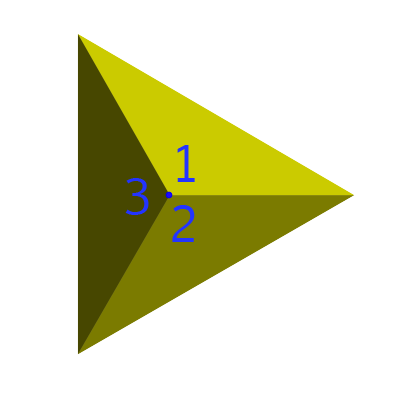At least 3 faces have to meet at every vertex.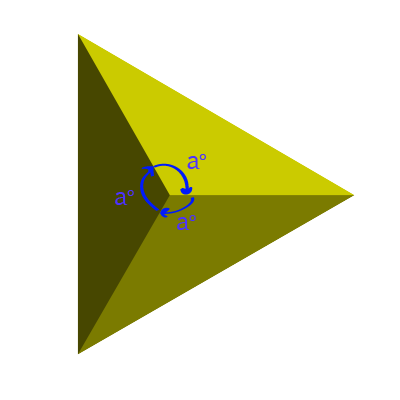The interior angles of the faces where they meet at each vertex must add up to less than $360^\circ$. When all the angles add up to $360^\circ$, the vertex flattens out.

As all the faces of a Platonic Solid must be the same regular polygon, these facts limit the options.

Let's explore the possibilities: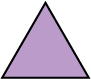The interior angles of a regular (equilateral) triangle are $60^\circ$. So our solid can have:

• 3 triangles meeting at each vertex ($3 \times 60 = 180^\circ$)
• 4 triangles meeting at each vertex ($4 \times 60 = 240^\circ$)
• 5 triangles meeting at each vertex ($5 \times 60 = 300^\circ$)
There can't be any more: the solid flattens out at an angle sum of $360^\circ$The interior angles of a regular quadrilateral (square) are $90^\circ$. So our solid can have:

• 3 quadrilaterals meeting at each vertex ($3 \times 90 = 270^\circ$)
There can't be any more: the solid flattens out at an angle sum of $360^\circ$.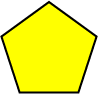The interior angles of a regular pentagon are $108^\circ$. So our solid can have:

• 3 pentagons meeting at each vertex ($3 \times 108 = 324^\circ$)
There can't be any more: the solid flattens out at an angle sum of $360^\circ$.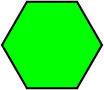The interior angles of a regular hexagon are $120^\circ$. But $3 \times 120 = 360^\circ$, so we can't build a solid with hexagons as it will flatten out.

We've found 5 different possibilities for the faces meeting at each vertex. So there are 5 possible different solids. Here they are:

Faces Meeting at Each Vertex Angle Sum at Vertex Picture Name of Solid
3 Equilateral Triangles $180^\circ$Tetrahedron
4 Equilateral Triangles $240^\circ$Octahedron
5 Equilateral Triangles $300^\circ$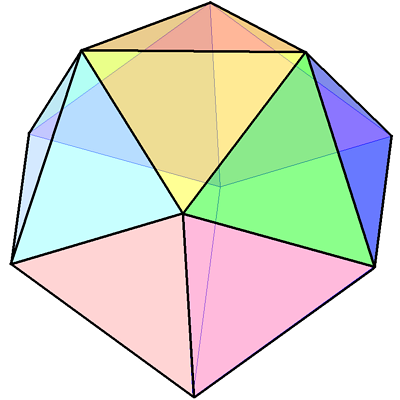Icosahedron
3 Squares $270^\circ$Cube (or Hexahedron)
3 Pentagons $324^\circ$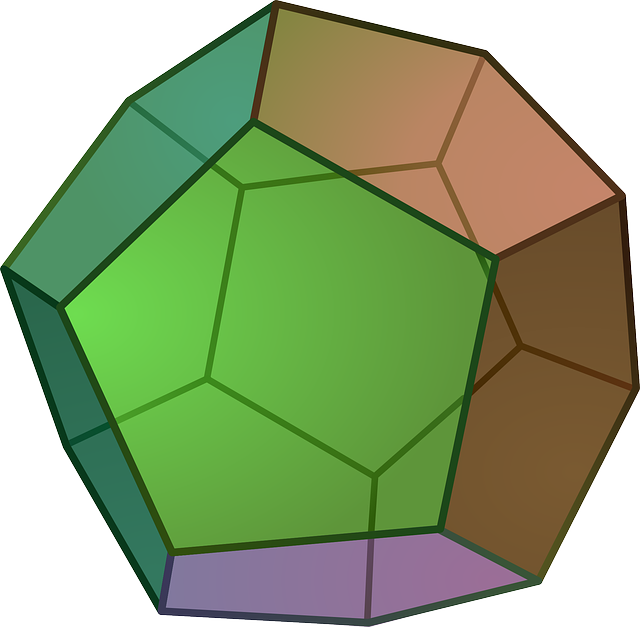Dodecahedron

## An Alternative Explanation Using Euler's Formula

Euler's formula is a result that works for convex polyhedra (ones without dents). It states that the number of faces plus the number of vertices minus the number of edges must equal $2$:

$F + V - E = 2$

The Platonic solids are convex polyhedra, so they must satisfy Euler's formula.

### ExampleA tetrahedron has 4 faces, 4 vertices and 6 edges. So:

$4 + 4 - 6 = 2$
and Euler's formula works for the tetrahedron.

We can see why Euler's formula works by adding a vertex or an edge to a tetrahedron: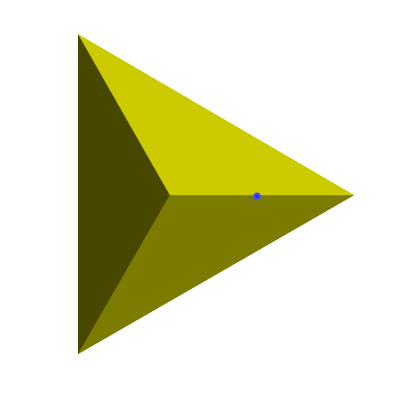If we add an extra vertex (in the middle of one of the edges, say), then we also add an extra edge. So,

$4 + 5 - 7 = 2$
again, and Euler's formula still works.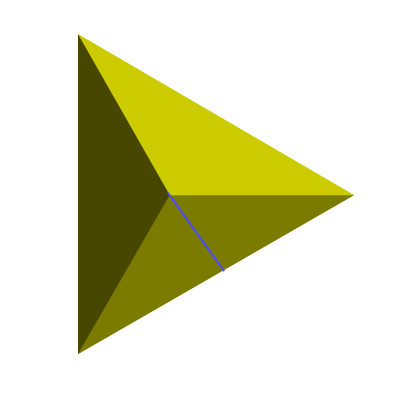If we add an extra edge (cutting one face in half, say), then we also add an extra face. So,

$5 + 4 - 7 = 2$
again, and Euler's formula still works.

No matter what we do to our solid, Euler's formula continues to give us $2$. You can read more about Euler's formula in the article on Euler's formula.

## So, How Does Euler's Formula Show Us There are Only Five Platonic Solids

• All faces have the same number of edges. Let's call this $n$.
• The same number of faces meet at each vertex. Let's call this $c$. It's also equal to the number of edges that meet at each vertex.

It turns out that the numbers $n$ and $c$ actually are enough to tell us which Platonic solid we are dealing with.

If the number of faces in the solid is $F$, and each face has $n$ edges, then if we pull the solid apart so that each face lies flat, we will end up with $nF$ edges:Of course, in the solid, each of these edges is shared by two faces. So, if the solid has a total of $E$ edges, we know that

$nF = 2E$

Now let's deal with the vertices. When we pull our solid apart, each vertex is split into bits: one bit for each face that meets at the vertex. Let's see this happening on our tetrahedron: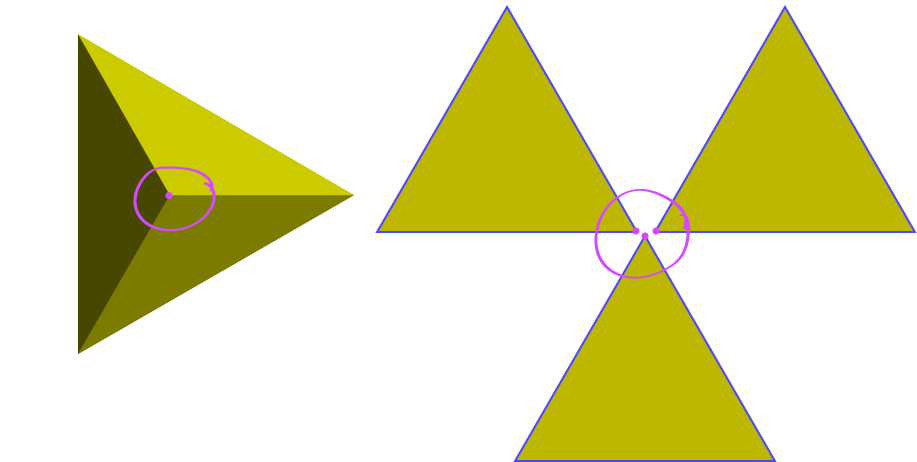As 3 faces meet at each vertex in a tetrahedron, each vertex splits into 3. So, if $c$ faces meet at each vertex, our pulled apart Platonic solid has $cV$ vertices, where $V$ is the number of vertices in the original solid.

The faces of our pulled apart solid now form a collection of identical polygons, so the total number of edges is equal to the total number of vertices in the pulled apart solid. This means that

$cV = 2E$

### So, where does Euler's Formula Come Into It?

Let's rearrange our equations to get $F$ and $V$ in terms of $E$:

\begin{align*} nF &= 2E\\ F &= \dfrac{2E}{n}\\ cV &= 2E\\ V &= \dfrac{2E}{c} \end{align*}
Now, plugging these into Euler's formula gives:
\begin{align*} F + V - E &= 2\\ \dfrac{2E}{n} + \dfrac{2E}{c} - E &= 2 \end{align*}
Dividing everything by $2E$ yields
\begin{align*} \dfrac{1}{n} + \dfrac{1}{c} - \dfrac{1}{2} &= \dfrac{1}{E}\\ \end{align*}
Since the number of edges (E) must be positive, we have
\begin{align*} \dfrac{1}{n} + \dfrac{1}{c} - \dfrac{1}{2} &> 0\\ \dfrac{1}{n} + \dfrac{1}{c} &> \dfrac{1}{2} \end{align*}

Now let's see what values of $n$ and $c$ will work:

n c $\dfrac{1}{n} + \dfrac{1}{c}$ $> \dfrac{1}{2}$? Picture Name of Solid
3 3 0.66666.... YesTetrahedron
3 4 0.583.... YesOctahedron
3 5 0.533.... YesIcosahedron
4 3 0.583.... YesCube (or Hexahedron)
4 4 0.5 No No Solid No Solid
5 3 0.533.... YesDodecahedron
5 4 0.45 No No Solid No Solid

And nothing else will work. So, there are only five Platonic Solids.

Note: As I said earlier, the values of $n$ and $c$ are enough to tell you what solid you have. Together they form the Schläfli symbol for the polyhedron. Here are the Schläfli symbols for the Platonic solids:

• Tetrahedron: $\{3,3\}$
• Octahedron: $\{3,4\}$
• Icosahedron: $\{3,5\}$
• Cube: $\{4,3\}$
• Dodecahedron: $\{5,3\}$

### Description

There are several lessons related to 3D geometry such as

1. Euler's formula
2. Vertices, Edges and Faces
3. Volumes of 3D shapes
4. etc

Even though we've titled this lesson series to be more inclined for Year 10 or higher students, however, these lessons can be read and utilized by lower grades students.

### Prerequisites

Understanding of 3D shapes

### Audience

Year 10 or higher, but suitable for Year 8+ students

### Learning Objectives

Get to know 3D Geometry

Author: Subject Coach
You must be logged in as Student to ask a Question.